## 浅谈6.6万哈弗M6的三大“看点”，让你不再“沉默”

2019年08月19日 17:15 来源：车行天下 超过：13817次关注

《沉默的证人》在8月16日正式上映了，这部影片应该是新型的一种港片，导演是外国的，演员用的是大陆流量花和香港班底，而故事是非常传统的的港片动作片，其一经播出，就获得了众多影迷的一致好评。而在汽车领域，也有一款车型备受消费者青睐，就是哈弗旗下的哈弗M6，其全面升级归来，拥有时尚的外观、科技的配置等，同时，还为消费者带来了超级优惠的购车价格和政策。因此，许多车主都强烈推荐，今天我们就看看哈弗M6车主的真实用车感受吧!智能安全 一路保驾护航

对于新婚夫妇来说，婚后的生活不再是单打独斗，而是两个人携手同行，每天饭桌前等待另一半安全归来的焦虑，上下班时有人担心你高峰拥堵的小碰撞，出差在外时有人担心你长途开车种种意外，一辆家用车辆承载了家人所有的担心与守候。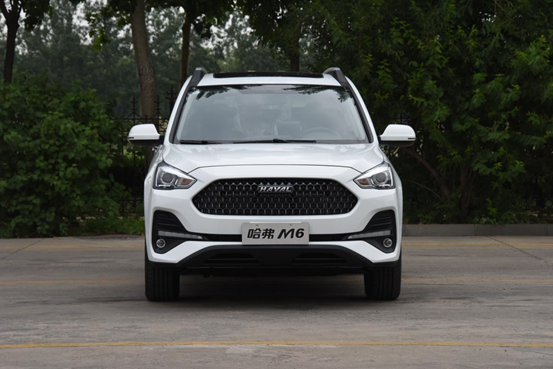哈弗M6年轻车主欧阳先生是一名快车司机，新婚不久，生活压力不是很大，但每天也是早出晚归为自己的小家庭努力奋斗着。在城市的车水马龙中穿梭，妻子最担心的就是丈夫的安全，哈弗M6的高刚性笼式车身设计，关键部位钢板厚度达2mm，车辆抗冲击能力大大加强，车身关键部位采用激光拼焊技术打造，提高整车刚度、强度有效保护成员安全，等于为车辆披上了高强度铠甲。在智能安全方面哈弗M6提供了非常全面的防护，比如ESP车身稳定系统、TPMS智能胎压监测系统、倒车影像、TCS牵引力控制系统、HHC、HDC、RMI防侧翻系统等周全配备，一路保驾护航，让意外无可乘之机。

越级配置 乐趣无处不在

哈弗M6的另一位车主邓先生今年孩子刚上幼儿园，家中有个刚上幼儿园的孩子是什么体验?在家时家用物品乱飞，外出时如脱缰魔王，幼儿园阶段的孩子正处于认识世界的阶段，对世界充满好奇，这个阶段孩子的家庭最需要拥有一辆高配置哈弗M6，让孩子在家长的陪伴下享受科技带来的乐趣。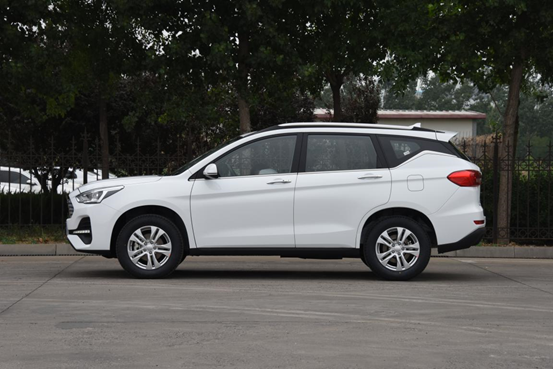邓先生非常注重孩子的世界观的培养，每逢周末或者节假日就会全家一起去参观博物馆、科技馆，也会带着孩子去生态区去认识大自然。去郊外的路上，打开智能双模防夹电动天窗，将车辆进行定速巡航，全家一起享受大自然的风光。即使是夏天孩子在车中也不会闹，打开同级独有的双温区自动恒温空调，根据孩子需要调整好后排座椅的角度，留出足够的玩耍空间，一个凉爽的车内儿童房就搭建好了，真的是降服家中小魔王，一辆哈弗M6就够了。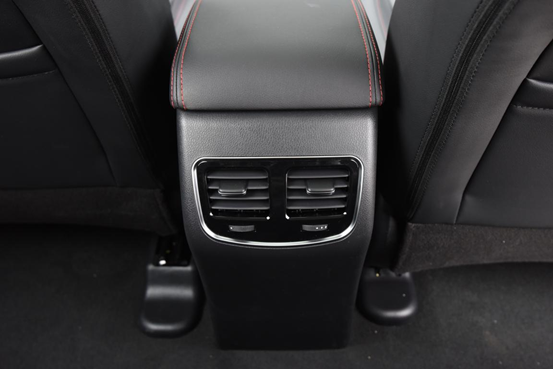超大空间 看得见的便利

在空间方面，邓先生反应，哈弗M6的空间真的超级大，无论家人一块出去郊游、孩子上下学接送以及周末带孩子游乐场陪玩都能轻松应对，哈弗M6不仅方便了自己的生活，还提升了幸福感。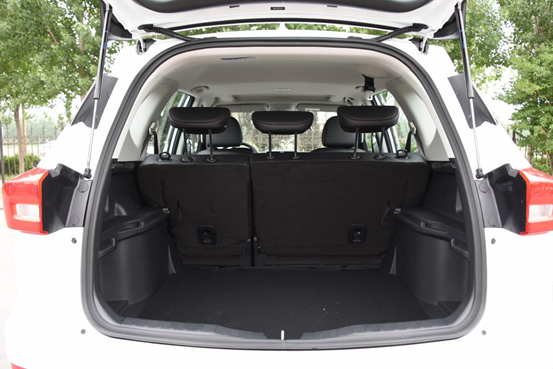除此之外，有时候妻子购买了家居大物件，也不需要找朋友或者搬家公司，只需要调整下哈弗M6的后排座椅就能轻松将容积808L的后备厢升级成2010L的超大空间，省钱的同时还省下了许多人情债。哈弗M6超大空间给家庭带来看得见的便利和生活质量的提升，非常值得拥有。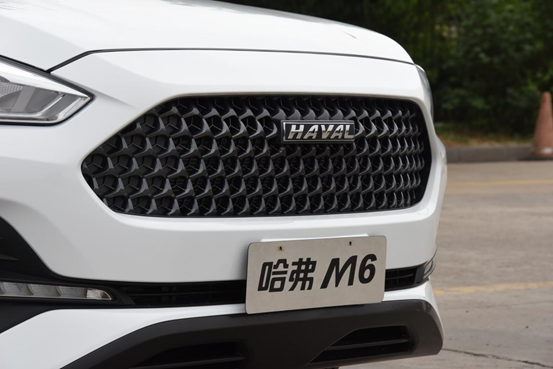小结

哈弗M6已经不仅仅只是我们出行的工具，它可以给我们最真诚的陪伴，最舒适的驾趣体验。另外，6.6万哈弗M6还推出了“1+5=6”的优惠政策，即首付1.5万就可将其带回家，还可以享受五年超长贷期，月供仅1290元。在排放标准问题上，哈弗M6国五、国六版均已上市。这样的优质好车，快点“享受”起来!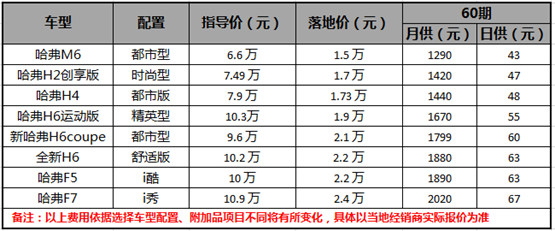#### 相关文章

0-500 字已有评论 0条 查看评论>>

### 热门标签

﻿
• 快速找车
• 选择品牌
• 选择品牌
• A  奥迪
• A  阿斯顿·马丁
• A  阿尔法·罗密欧
• B  宝沃
• B  布加迪
• B  巴博斯
• B  保时捷
• B  宾利
• B  奔驰
• B  宝马
• B  本田
• B  别克
• B  标致
• B  比亚迪
• B  宝骏
• B  北汽制造
• B  北汽新能源
• B  北汽幻速
• B  北汽威旺
• B  北京汽车
• B  奔腾
• B  北汽绅宝
• C  长安
• C  长安商用
• C  长城
• C  昌河
• D  大众
• D  道奇
• D  DS
• D  东南
• D  东风风神
• D  东风风行
• D  东风小康
• D  东风风度
• D  东风
• F  福特
• F  丰田
• F  菲亚特
• F  法拉利
• F  福田
• F  福迪
• F  福汽启腾
• G  观致
• G  广汽传祺
• G  广汽吉奥
• G  GMC
• H  红旗
• H  汉腾汽车
• H  哈弗
• H  哈飞
• H  海格
• H  海马
• H  华颂
• H  黄海
• H  华泰
• H  恒天
• J  吉利汽车
• J  捷豹
• J  Jeep
• J  江淮
• J  江铃
• J  金杯
• J  九龙
• J  金旅
• K  凯翼
• K  凯迪拉克
• K  克莱斯勒
• K  科尼塞克
• K  卡威
• K  开瑞
• L  路虎
• L  林肯
• L  劳斯莱斯
• L  兰博基尼
• L  雷克萨斯
• L  铃木
• L  雷诺
• L  理念
• L  力帆
• L  莲花汽车
• L  猎豹
• L  路特斯
• L  陆风
• M  马自达
• M  MG
• M  MINI
• M  玛莎拉蒂
• M  摩根
• M  迈凯轮
• N  纳智捷
• O  欧宝
• O  讴歌
• O  欧朗
• Q  奇瑞
• Q  起亚
• Q  启辰
• R  日产
• R  荣威
• R  瑞麒
• S  三菱
• S  斯威汽车
• S  萨博
• S  smart
• S  斯柯达
• S  斯巴鲁
• S  思铭
• S  双龙
• S  上汽大通
• S  双环
• T  特斯拉
• T  腾势
• W  沃尔沃
• W  五菱汽车
• W  五十铃
• W  威兹曼
• W  威麟
• X  现代
• X  雪佛兰
• X  雪铁龙
• X  西雅特
• Y  一汽
• Y  英菲尼迪
• Y  英致
• Y  依维柯
• Y  野马汽车
• Y  永源
• Z  众泰
• Z  中华
• Z  中兴
• Z  知豆
• 选择车系
• 选择车系
• 车型对比
• 选择品牌
• 选择品牌
• A  奥迪
• A  阿斯顿·马丁
• A  阿尔法·罗密欧
• B  宝沃
• B  布加迪
• B  巴博斯
• B  保时捷
• B  宾利
• B  奔驰
• B  宝马
• B  本田
• B  别克
• B  标致
• B  比亚迪
• B  宝骏
• B  北汽制造
• B  北汽新能源
• B  北汽幻速
• B  北汽威旺
• B  北京汽车
• B  奔腾
• B  北汽绅宝
• C  长安
• C  长安商用
• C  长城
• C  昌河
• D  大众
• D  道奇
• D  DS
• D  东南
• D  东风风神
• D  东风风行
• D  东风小康
• D  东风风度
• D  东风
• F  福特
• F  丰田
• F  菲亚特
• F  法拉利
• F  福田
• F  福迪
• F  福汽启腾
• G  观致
• G  广汽传祺
• G  广汽吉奥
• G  GMC
• H  红旗
• H  汉腾汽车
• H  哈弗
• H  哈飞
• H  海格
• H  海马
• H  华颂
• H  黄海
• H  华泰
• H  恒天
• J  吉利汽车
• J  捷豹
• J  Jeep
• J  江淮
• J  江铃
• J  金杯
• J  九龙
• J  金旅
• K  凯翼
• K  凯迪拉克
• K  克莱斯勒
• K  科尼塞克
• K  卡威
• K  开瑞
• L  路虎
• L  林肯
• L  劳斯莱斯
• L  兰博基尼
• L  雷克萨斯
• L  铃木
• L  雷诺
• L  理念
• L  力帆
• L  莲花汽车
• L  猎豹
• L  路特斯
• L  陆风
• M  马自达
• M  MG
• M  MINI
• M  玛莎拉蒂
• M  摩根
• M  迈凯轮
• N  纳智捷
• O  欧宝
• O  讴歌
• O  欧朗
• Q  奇瑞
• Q  起亚
• Q  启辰
• R  日产
• R  荣威
• R  瑞麒
• S  三菱
• S  斯威汽车
• S  萨博
• S  smart
• S  斯柯达
• S  斯巴鲁
• S  思铭
• S  双龙
• S  上汽大通
• S  双环
• T  特斯拉
• T  腾势
• W  沃尔沃
• W  五菱汽车
• W  五十铃
• W  威兹曼
• W  威麟
• X  现代
• X  雪佛兰
• X  雪铁龙
• X  西雅特
• Y  一汽
• Y  英菲尼迪
• Y  英致
• Y  依维柯
• Y  野马汽车
• Y  永源
• Z  众泰
• Z  中华
• Z  中兴
• Z  知豆
• 选择车系
• 选择车系
• 选择车型
• 选择车型
• 意见反馈